# 1455D - Sequence and Swaps

Contest: Educational Codeforces Round 99Photo by Leopold Stenger on Unsplash

This article is about the thinking process and solution of a question from Educational Codeforces Round 99. Here’s the link to the question.

# Problem —

You are given a sequence A consisting of n integers [a1,a2,…,an] and an integer x. Your task is to sort the sequence in non-decreasing order.

For sorting, you are allowed to perform the following operation any number of times(possibly zero):
Choose an integer i such that 1 ≤ i ≤ n and ai > x (i.e., the ith integer in sequence A is strictly greater than x), and swap the values of ai and x.

For instance, if a = [0, 2, 3, 5, 4], x = 1, the following sequence of operation is possible:

1. choose i=2 (it is possible since a2 > x), then a=[0,1,3,5,4], x = 2;
2. choose i=3 (it is possible since a3 > x), then a=[0,1,2,5,4], x = 3;
3. choose i=4 (it is possible since a4 > x), then a = [0,1,2,3,4], x = 5.

Calculate the minimum number of operations you have to perform so that A becomes sorted, or report that it is impossible.

# Solution —

By playing around a bit with the question, one could arrive at the following observations:

## Observations

1. Since x is always swapped with a value of the sequence A such that x< ai, x always increases.
2. The x divides the sequence in two parts —
[a1, …, ai, x, … an],
i. Left side of x, having values ≤ x;
ii. Right side of x, having values > x;
3. The sequence can only be sorted if the left side of x is completely sorted. If it’s not, then there is no way to sort the left side as the only permissible operation, swapping, occurs with the sequence on the right side of the x.

If the left side is sorted, then our task is reduced to sorting the right side.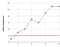x = 2 | Plotted on google sheets.

We can understand better by considering the above example. Here x = 2. From the above graph, we can conclude that the left side is sorted and the right side is not sorted because of the value 6.
To make the right side of the sequence (or upper part in the graph) sorted, all the values on the left of the value 6 (including 6) should be sorted. Since the value of x always increases, we can’t just swap x with any value other than the leftmost value on the right side. Because if we swap x with some value other than the leftmost value of the right side, the right side can no longer be sorted. The below graph shows what can happen when we swap the value of x with some value other than the leftmost value of the right side.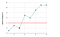Swapping x and a value other than the leftmost value of the right side. The initial value of x was 2; the Leftmost value was 3, the value swapped with x was 6, the final value of x = 6, and the sequence 3, 2, 7 is not sorted.

Conclusion: To sort the sequence, we have to start swapping from the leftmost value of the right side, and simultaneously we also have to increment the number of steps required. On updating the value of x after swapping, there will be a new right portion.

Is that all? Not quite.

This isn’t completely correct, for we might count extra steps in cases when the right portion is already sorted.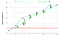Even though the sequence is already sorted, following our procedures, we will count extra steps.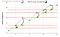Graph showing example of unnecessary swapping that will be counted

The above examples show that incrementing the number of steps every time we swap can give incorrect results. We can observe that only swaps before the condition: A[ind] < x really matters. The rest of the swaps don’t matter because they are operating on the sorted portion.

One more thing to note is that, on the right side, if a value is less than x, then we have to check if the previous value with which x has been swapped is less than or equal to the current value. If not, then the sequence cannot be sorted. For example, have a look at the below graph.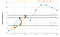3, 6, 5 is unsorted. The value 5 will not be swapped with any other value as x = 7 which is greater than the value 5, thus the sequence can no longer be sorted.

Keeping the above points in mind, here’s how we can solve this question —

• Find an index in A having value greater than equal to x, also make sure that all the elements before this are sorted in non-decreasing order; if that’s not the case, then the sequence cannot be sorted.
• Maintain two variables, say finalAns and ans; ans will be updated every time we swap, and finalAns will be updated when we arrive at condition A[ind] < x;
• If the left side is in sorted order, start traversing the sequence from the above found index. If A[ind] < val (where val is the previous value with which x was swapped), then the sequence cannot be sorted. Otherwise, if A[ind] > x, swap their values and increment the total steps by 1. Also, make sure you are storing the previous value with which x was swapped.
If A[ind] < x, then finalAns = finalAns + ans and ans = 0;

The time complexity of this approach is O(N).

I hope it makes sense. Here’s the link to my solution.

Love sharing new things I learn🌺